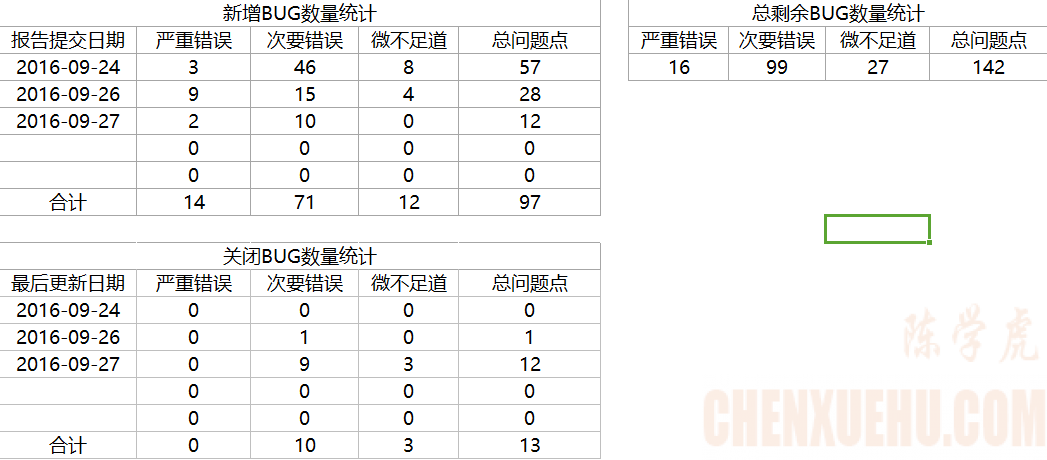# BUG数据自动统计，基于XML和EXCEL1445

263

2016 年 09 月 27 日20:34:59 1 1,219 ℃ 1019字阅读3分23秒

### 1、本轮次提交的BUG数量：

COUNTIFS(DATA!J:J,"="&B4,DATA!F:F,"=严重错误")

COUNTIFS(DATA!J:J,"="&B4,DATA!F:F,"=次要错误")

COUNTIFS(DATA!J:J,"="&B4,DATA!F:F,"=微不足道")

### 2、本轮测试关闭的BUG数量

COUNTIFS(DATA!P:P,"="&B13,DATA!R:R,"=已关闭",DATA!F:F,"=严重错误")

COUNTIFS(DATA!P:P,"="&B13,DATA!R:R,"=已关闭",DATA!F:F,"=次要错误")

COUNTIFS(DATA!P:P,"="&B13,DATA!R:R,"=已关闭",DATA!F:F,"=微不足道")

### 3、总剩余BUG数量

COUNTIFS(DATA!R:R,"<>已关闭",DATA!F:F,"=严重错误")

COUNTIFS(DATA!R:R,"<>已关闭",DATA!F:F,"=次要错误")

COUNTIFS(DATA!R:R,"<>已关闭",DATA!F:F,"=微不足道")Happly

##### 历史上的今天

27
• 免费优惠券
• 免费领取优惠券
•• 支付宝红包领取
• 支付宝大额红包
•• 版权声明：本文为原创文章，转载请附上原文出处链接及本声明。
• 转载请注明：BUG数据自动统计，基于XML和EXCEL | https://chenxuehu.com/article/2016/09/5660.html